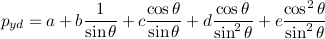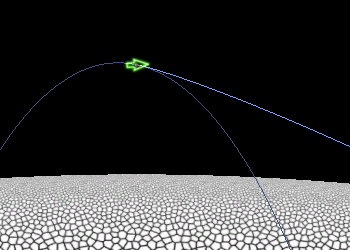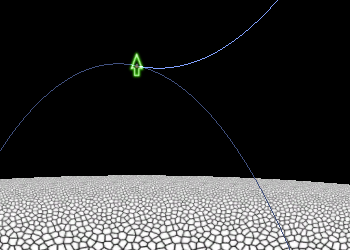## Monday, December 3, 2007

### Powered trajectory plot

Soonest-descent trajectory

I've spent a bit of time attempting to simplify the math for the soonest-descent trajectory angle. The basic equation relates the rocket angle (which is presumed to be held constant during the horizontal braking phase) to the difference between the height when horizontal braking finishes and the height at which vertical braking must start.

The equation ends up having the sine of the rocket angle in its denominators, which means it gets squirrelly near zero. However, as you can see in the last plot from two weeks ago, the curve does not look complicated at all, and it looks well-behaved at zero.

It turns out that the equation can be simplified down to this form:If that was all we knew about the equation, we'd be stuck. However, it turns out that the constants b and c will always have opposite signs. Likewise d and e will have opposite signs. This means that their respective terms cancel each other out as the angle approaches zero. I figured this out by writing out the series expansions for the sines and cosines.

On a separate note, I put a very simple solver for the equation into my lander game and draw an arrow indicating the direction to fire the rocket for soonest descent. It turns out that, if horizontal braking finishes at the same instant that vertical braking starts (which is what the equation is aiming for), there's often quite a large instantaneous turn necessary. This is hard to fly. If I was intending to produce a flyable trajectory I'd need to add in sufficient time for the rocket to turn upright before commencing vertical braking.

Powered trajectory plot

The ballistic trajectory plot has turned out to be very useful. It shows you where the rocket will go if you don't do anything. I got to thinking that perhaps a similar plot for powered flight might be useful. The idea is to show where the rocket will go if you keep the rocket firing continuously but don't do any additional steering. (My rocket attitude is defined in screen space, which rotates to keep the rocket at the top of the screen, so technically the rocket does rotate when you don't do anything. This turned out to be the most useful frame of reference for controlling rocket direction.)

The powered trajectory plot allows you to swivel the rocket and experimentally find the horizontal braking trajectory that I was aiming for in the section above. Here's a sample:I may cut off the trajectory plot when (local) horizontal velocity reaches zero. It would also be good to mark that point with a circle or something so it's clear. Next, I plan to compute the height at which vertical braking must start at the point where horizontal velocity reaches zero. This could be color-coded based on whether it's above or below the height where the rocket stop moving horizontally. Using this you can visually solve the equation to figure out how to fly an approach trajectory.

Scott said...

Ah, very cool.

I ran into a similar problem (though much simpler) for the autopilot in the space game I was prototyping. Given any starting position and velocity, it could fly you to any other object (matching its velocity) with the thrusters always maxed out. I even had it take into account damaged and unbalanced thruster pairs.

The one issue I could never figure out is how to do the timing to allow the ship to turn. The autopilot needed a bit of human intervention to make the best time by turning the ship around to use the main engine as much as possible. I had a heuristic that worked okay when the relative starting velocities were zero, but failed pretty badly otherwise.

James McNeill said...

Very interesting-sounding! I'd love to know more details about what you were doing. The first part sounds a bit like a space pathfinding problem I was working on earlier this year. Is it at all like that?